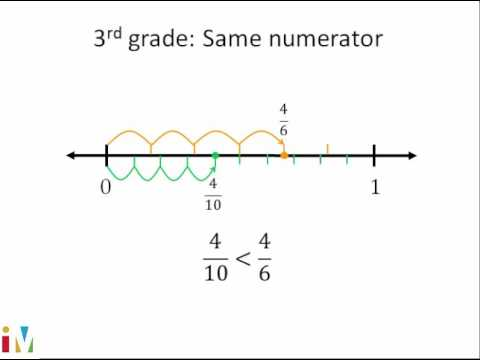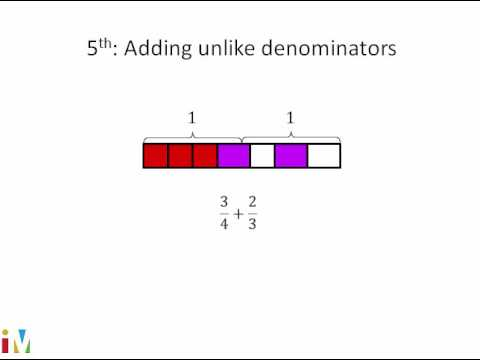Video

# Intro to subtracting mixed numbers (Full video)

Description: Sal subtracts 2 mixed numbers with common (like) denominators. Let's get some practice subtracting mixed numbers. Well, we can rewrite this, we could write the numbers on top of each other, we could say this is two, in that magenta color, say this is 2 5/8, actually let me write it a little bit further spaced apart, 2 5/8, minus 1 2/8. So this is going to be equal to one and three eighths.

### Other videos you might be interested in### Comparing Fractions

#### Illustrative Mathematics